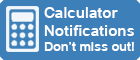Calculator Library
Free Online CalculatorsHome » 87 Calculators » Other » Statistical Calculator

Huge Domain Name Sale | Free Domains
Domain names on sale at crazy prices. Register a new domain for FREE when you buy another service.

## STATISTICAL CALCULATOR

INSTRUCTIONS

You can use this calculator to analyse statistical data. It will calculate mean, median, mode, standard deviation, range and more from a set of data.

Simply enter the data using the examples below then click the "calculate" button.

Enter Data Here
 Title (Optional) Statistical Data Example Data Entry 371629.518 OR 37 16 29.5 18 Format data with spaces between values

#### NOTES

This calculator returns the following values for a data set:

• Maximum - The highest value in the data set.
• Minimum - The lowest value in the data set.
• Sum - The addition of all values in the data set.
• Mean - The addition of all values divided by the total number of values.
• Median - The middle value in the data set.
• Range - The difference between the lowest and highest values.
• Midrange - The mean of the maximum and minimum values in the data set.
• Standard Deviation - The amount of variation from the mean value in the data set.
• Percentage Unique - The percentage of values in the data set that are unique.